## 1.单链表的定义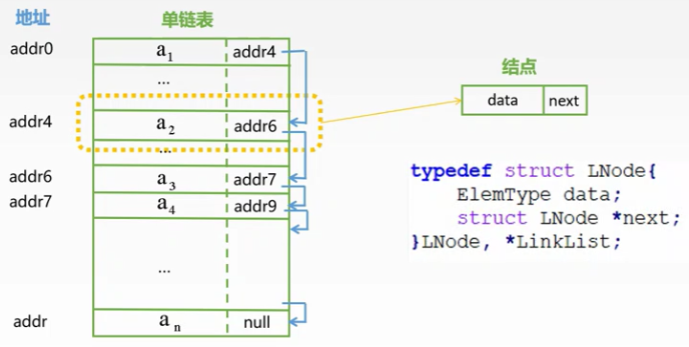• 无头结点的单链表
• 有头节点的单链表

• 链表的第一个位置和其他位置的操作统一(比如插入操作：无头节点的链表，在表中插入结点的时候两边都有结点，而在表头插入结点的时候左边是没有结点的，而有头节点就都是一样的。)
• 空表和非空表的操作统一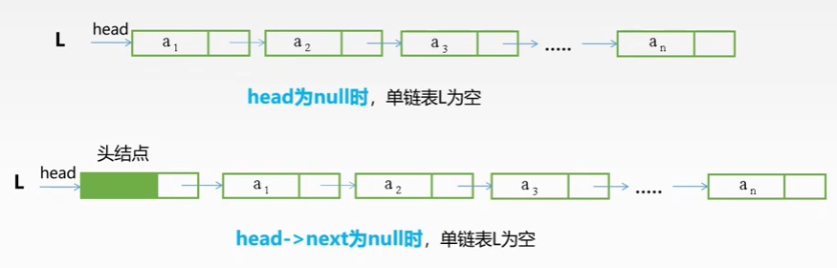## 2.单链表的基本操作

### 2.1头插法建立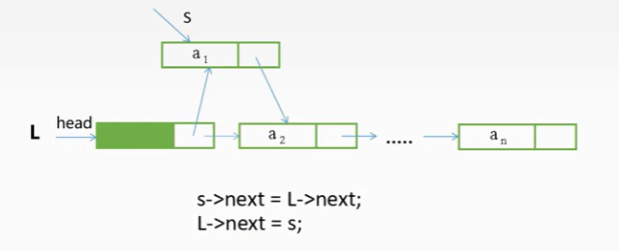//头插法
LNode *s;
int x;
L->next=NULL;
scanf("%d",&x);
while(x!=9999){
s=(LNode*)malloc(sizeof(LNode));
s->data=x;
s->next=L->next;
L->next=s;
scanf("%d",&x);
}
return L;
}### 2.2尾插法建立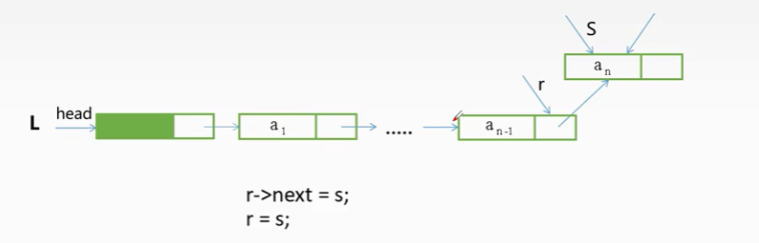LinkList List_TailInsert (LinkList &L){
int x;
LNode *s,*r=L; //注意这里重新定义一个指针r，作为尾指针，同时初始化为了头节点
scanf("%d",&x);
while(x!=999){
s=(LNode*)malloc(sizeof(LNode));
s->data=x;
r->next=s;
r=s; //修改尾指针，指向新插入的结点
scanf("%d",&x);
}
r->next=NULL;
return L;
}


### 2.3按序号查找&按值查找

LNode *GetElem(LinkList L,int i){
int j=1; //标识当前结点的序号
LNode *p=L->next; //当前所查找的结点，初始化为头节点的下一个结点，因为头节点不保存数据
if(i==0){ //序号不合法
return L;
}
if(i<1){ //序号不合法
return NULL;
}
while(p&&j<i){ //当结点不为空，且序号小于j的时候,继续循环
p=p->next;
j++;
}
return p;
}


LNode *LocateElem(LinkList L,ElemType e){
//初始化一个指针指向头节点的下一个结点
LNode *p=L->next;
//判断结点不为空，且数据不为e
while(p!=NULL&&p->data!=e){
//如果结点不为空，且值不为e，则指针继续向下移动
p=p->next;
}
return p;
}


### 2.4插入结点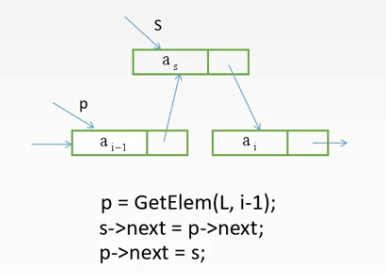注意 下面的代码，不能交换位置。

s->next=p->next;
p->next=s;


why???

p->next=s;这时已经将p结点中存储的i结点的地址给覆盖了，成了新结点的地址，接着再s->next=p->next;这相当于讲s结点的指针指向了他自己。这样就把后面的链表给丢弃了。

### 2.5删除结点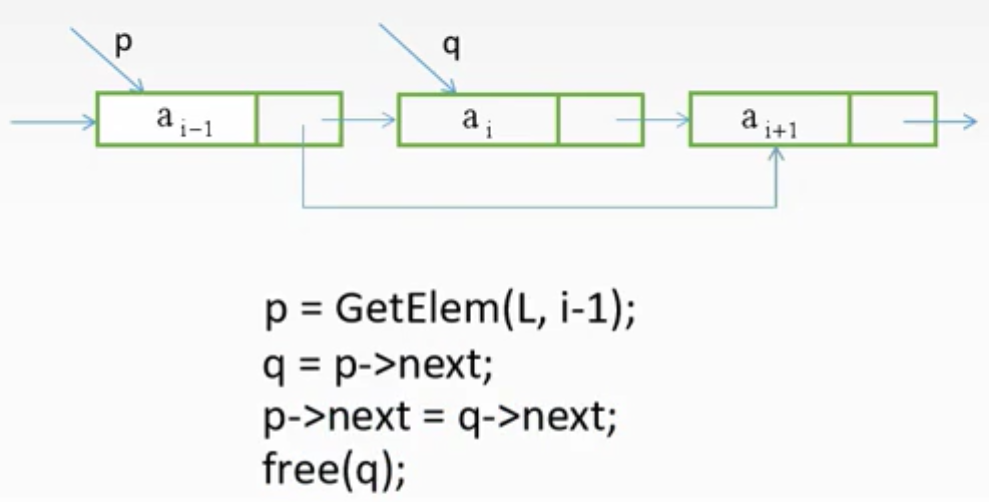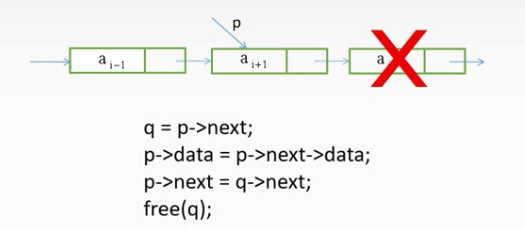### 2.6求表长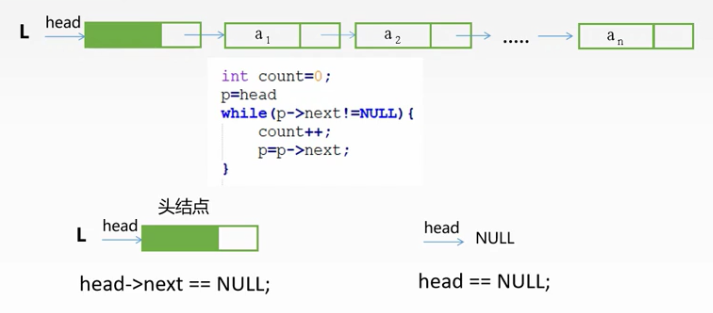## 3.特殊链表

### 3.1双链表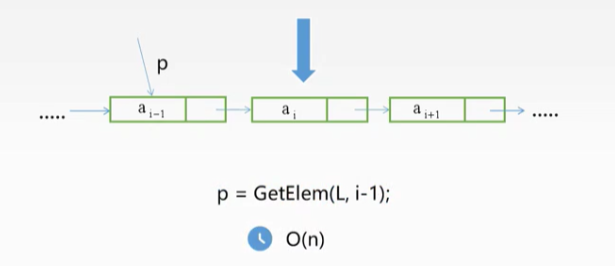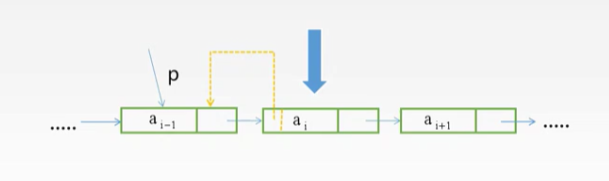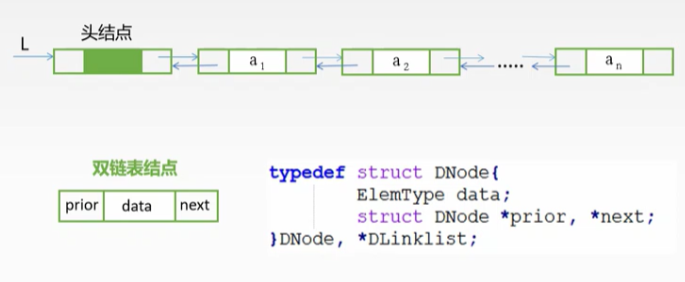### 3.1双链表插入操作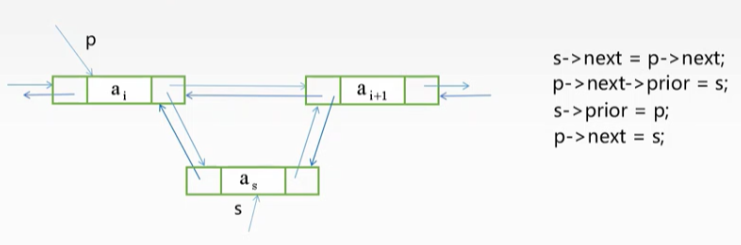### 3.1双链表删除操作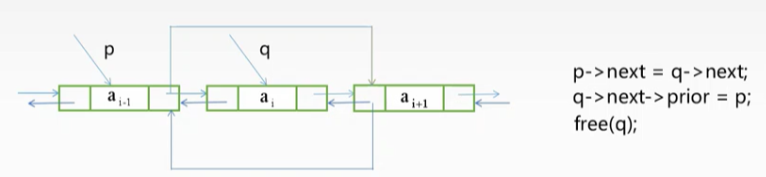### 3.2循环链表

#### 3.2.1循环单链表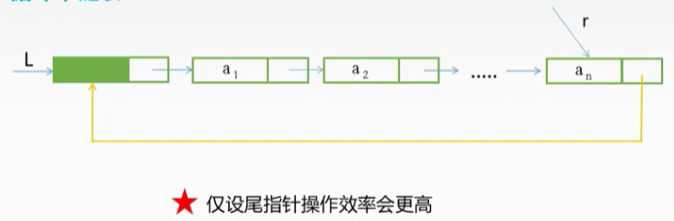#### 3.2.3循环链表判空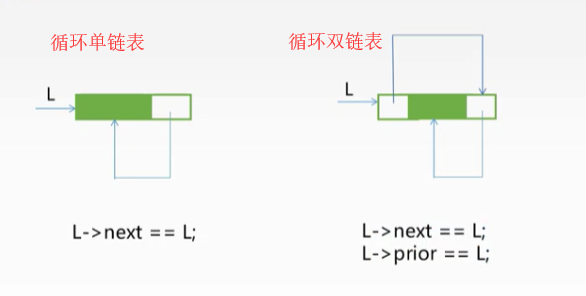### 3.3静态链表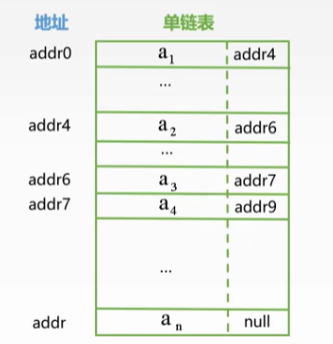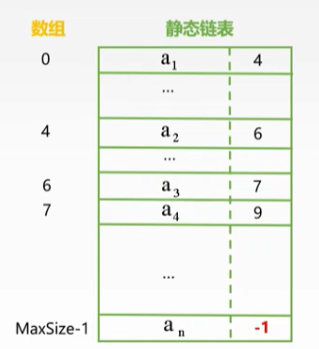#define MaxSize 50
typedef struct DNode{
ElemType data;
int next;

#### 欢迎关注公众号 理木客 更多精彩等你发现